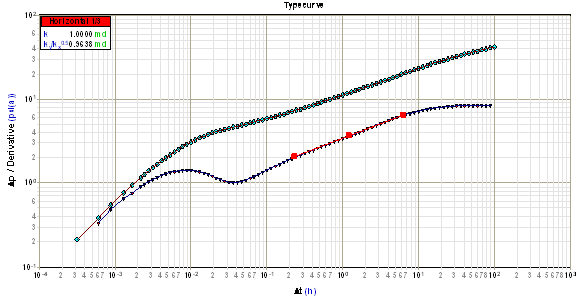# Elliptical Flow Analysis

In a horizontal well, the elliptical flow regime is often seen instead of, or immediately following, the linear horizontal flow regime.

The elliptical flow regime exhibits a slope of 1/3 on a log-log plot as shown below.The position of this line is used to calculate the square root of the horizontal permeability ratio (sqrt(ky / kx)) as follows:

The semi-log pressure derivative during the elliptical flow period in dimensionless form is:In dimensional parameters, for an oil reservoir, the semi-log pressure derivative becomes:Where:The horizontal permeability (kxy) is obtained from the horizontal radial analysis.  Alternatively, kxy may be obtained using k from a previous radial flow analysis for a vertical well in the same reservoir.

Upon re-arranging the second equation shown above, the square root of the horizontal permeability ratio can be expressed as follows:

For oil and water:For gas:Where:To calculate the square root of the horizontal permeability ratio, the above equations are used along with a Dpw' or Dyw' value read from the 1/3 slope line on the log-log plot at a corresponding Dt value. kxy is obtained from the horizontal radial analysis line.

## References

"Determination of Horizontal Permeability Anisotropy from Horizontal Well Tests", M.B. Issaka, K. Zaoral, A.K. Ambastha, L. Mattar, Paper presented at the 2000 SPE Saudi Arabia Section Technical Symposium, Dahran, Saudi Arabia, October 21 - 23.